# Bubble Sort Algorithm | Example | Time Complexity

## Bubble Sort-

• Bubble sort is the easiest sorting algorithm to implement.
• It is inspired by observing the behavior of air bubbles over foam.
• It is an in-place sorting algorithm.
• It uses no auxiliary data structures (extra space) while sorting.

## How Bubble Sort Works?

• Bubble sort uses multiple passes (scans) through an array.
• In each pass, bubble sort compares the adjacent elements of the array.
• It then swaps the two elements if they are in the wrong order.
• In each pass, bubble sort places the next largest element to its proper position.
• In short, it bubbles down the largest element to its correct position.

## Bubble Sort Algorithm-

The bubble sort algorithm is given below-

```for(int pass=1 ; pass<=n-1 ; ++pass)     // Making passes through array
{
for(int i=0 ; i<=n-2 ; ++i)
{
if(A[i] > A[i+1])                // If adjacent elements are in wrong order
swap(i,i+1,A);               // Swap them
}
}

//swap function : Exchange elements from array A at position x,y

void swap(int x, int y, int[] A)
{
int temp = A[x];
A[x] = A[y];
A[y] = temp;
return ;
}

// pass : Variable to count the number of passes that are done till now
// n : Size of the array
// i : Variable to traverse the array A
// swap() : Function to swap two numbers from the array
// x,y : Indices of the array that needs to be swapped```

## Bubble Sort Example-

Consider the following array A-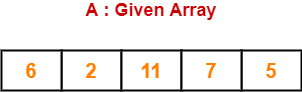Now, we shall implement the above bubble sort algorithm on this array.

### Step-01:

• We have pass=1 and i=0.
• We perform the comparison A > A and swaps if the 0th element is greater than the 1th element.
• Since 6 > 2, so we swap the two elements.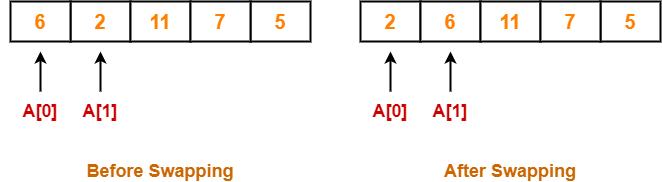### Step-02:

• We have pass=1 and i=1.
• We perform the comparison A > A and swaps if the 1th element is greater than the 2th element.
• Since 6 < 11, so no swapping is required.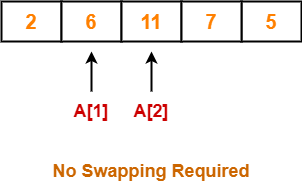### Step-03:

• We have pass=1 and i=2.
• We perform the comparison A > A and swaps if the 2nd element is greater than the 3rd element.
• Since 11 > 7, so we swap the two elements.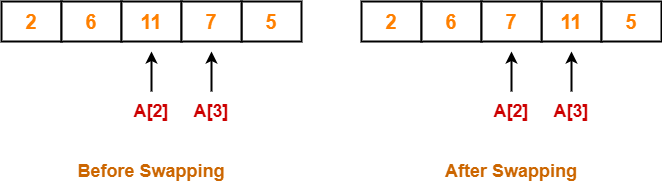### Step-04:

• We have pass=1 and i=3.
• We perform the comparison A > A and swaps if the 3rd element is greater than the 4th element.
• Since 11 > 5, so we swap the two elements.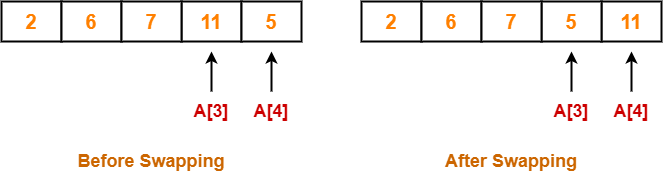Finally after the first pass, we see that the largest element 11 reaches its correct position.

### Step-05:

• Similarly after pass=2, element 7 reaches its correct position.
• The modified array after pass=2 is shown below-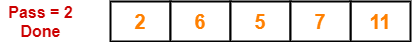### Step-06:

• Similarly after pass=3, element 6 reaches its correct position.
• The modified array after pass=3 is shown below-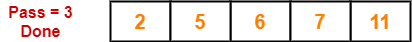### Step-07:

• No further improvement is done in pass=4.
• This is because at this point, elements 2 and 5 are already present at their correct positions.
• The loop terminates after pass=4.
• Finally, the array after pass=4 is shown below-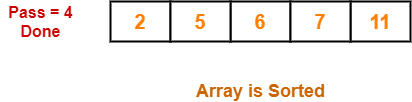## Optimization Of Bubble Sort Algorithm-

• If the array gets sorted after a few passes like one or two, then ideally the algorithm should terminate.
• But still the above algorithm executes the remaining passes which costs extra comparisons.

### Optimized Bubble Sort Algorithm-

The optimized bubble sort algorithm is shown below-

```for (int pass=1 ; pass<=n-1 ; ++pass)
{
flag=0                                // flag denotes are there any swaps done in pass

for (int i=0 ; i<=n-2 ; ++i)
{
if(A[i] > A[i+1])
{
swap(i,i+1,A);
flag=1                        // After swap, set flag to 1
}
}

if(flag == 0) break;                  // No swaps indicates we can terminate loop
}

void swap(int x, int y, int[] A)
{
int temp = A[x];
A[x] = A[y];
A[y] = temp;
return;
}```

### Explanation-

• To avoid extra comparisons, we maintain a flag variable.
• The flag variable helps to break the outer loop of passes after obtaining the sorted array.
• The initial value of the flag variable is set to 0.
• The zero value of flag variable denotes that we have not encountered any swaps.
• Once we need to swap adjacent values for correcting their wrong order, the value of flag variable is set to 1.
• If we encounter a pass where flag == 0, then it is safe to break the outer loop and declare the array is sorted.

## Time Complexity Analysis-

• Bubble sort uses two loops- inner loop and outer loop.
• The inner loop deterministically performs O(n) comparisons.

### Worst Case-

• In worst case, the outer loop runs O(n) times.
• Hence, the worst case time complexity of bubble sort is O(n x n) = O(n2).

### Best Case-

• In best case, the array is already sorted but still to check, bubble sort performs O(n) comparisons.
• Hence, the best case time complexity of bubble sort is O(n).

### Average Case-

• In average case, bubble sort may require (n/2) passes and O(n) comparisons for each pass.
• Hence, the average case time complexity of bubble sort is O(n/2 x n) = Θ(n2).

The following table summarizes the time complexities of bubble sort in each case-

 Time Complexity Best Case O(n) Average Case Θ(n2) Worst Case O(n2)

From here, it is clear that bubble sort is not at all efficient in terms of time complexity of its algorithm.

## Space Complexity Analysis-

• Bubble sort uses only a constant amount of extra space for variables like flag, i, n.
• Hence, the space complexity of bubble sort is O(1).
• It is an in-place sorting algorithm i.e. it modifies elements of the original array to sort the given array.

## Properties-

Some of the important properties of bubble sort algorithm are-

• Bubble sort is a stable sorting algorithm.
• Bubble sort is an in-place sorting algorithm.
• The worst case time complexity of bubble sort algorithm is O(n2).
• The space complexity of bubble sort algorithm is O(1).
• Number of swaps in bubble sort = Number of inversion pairs present in the given array.
• Bubble sort is beneficial when array elements are less and the array is nearly sorted.

## Problem-01:

The number of swapping needed to sort the numbers 8, 22, 7, 9, 31, 5, 13 in ascending order using bubble sort is-  (ISRO CS 2017)

1. 11
2. 12
3. 13
4. 10

## Solution-

In bubble sort, Number of swaps required = Number of inversion pairs.

Here, there are 10 inversion pairs present which are-

1. (8,7)
2. (22,7)
3. (22,9)
4. (8,5)
5. (22,5)
6. (7,5)
7. (9,5)
8. (31,5)
9. (22,13)
10. (31,13)

Thus, Option (D) is correct.

## Problem-02:

When will bubble sort take worst-case time complexity?

1. The array is sorted in ascending order.
2. The array is sorted in descending order.
3. Only the first half of the array is sorted.
4. Only the second half of the array is sorted.

## Solution-

• In bubble sort, Number of swaps required = Number of inversion pairs.
• When an array is sorted in descending order, the number of inversion pairs = n(n-1)/2 which is maximum for any permutation of array.

Thus, Option (B) is correct.

To gain better understanding about Bubble Sort Algorithm,

Watch this Video Lecture

Next Article- Insertion Sort

### Other Popular Sorting Algorithms-

Get more notes and other study material of Design and Analysis of Algorithms.

Watch video lectures by visiting our YouTube channel LearnVidFun.

SummaryArticle Name
Bubble Sort Algorithm | Example | Time Complexity
Description
Bubble Sort is the easiest sorting algorithm. Bubble Sort Algorithm with Example is given. The time complexity of Bubble Sort Algorithm is O(n2) and its space complexity is O(1).
Author
Publisher Name
Gate Vidyalay
Publisher Logo